Tamilnadu State Board New Syllabus Samacheer Kalvi 12th Business Maths Guide Pdf Chapter 7 Probability Distributions Ex 7.2 Text Book Back Questions and Answers, Notes.

## Tamilnadu Samacheer Kalvi 12th Business Maths Solutions Chapter 7 Probability Distributions Ex 7.2

Question 1.
Define possion distribution.
Solution:
Poisson distribution was derived in 1837 by a French Mathematician Simeon D. Poisson.
A random variable X is said to follow a Poisson distribution with parameter X if it assumes only non-negative values and its probability mass function is given by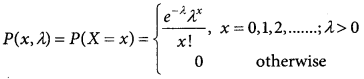Question 2.
Write any 2 examples for possion distribution
Solution:
Examples of Poisson distribution are given by

• The number of printing mistakes per page in a textbook.
• A number of lightning per second.
• The number of bacteria in one cubic centimetre.

Question 3.
Write the condition for which the Poisson distribution is limiting case of binomial distribution
Solution:
Poisson distribution is a limiting case of binomial distribution under the following conditions:
(i) n, the number of trials is indefinitely large i.e n → ∞
(ii) p, the constant probability of success in each trial is very small, i.e. p → 0.
(iii) np = λ is finite. Thus p = $$\frac { λ }{n}$$ and q = 1 – ($$\frac { λ }{n}$$)
where λ, is a positive real number.

Question 4.
Derive the mean and variance of the Poisson distribution.
Solution:
Derivation of Mean and variance of Poisson distribution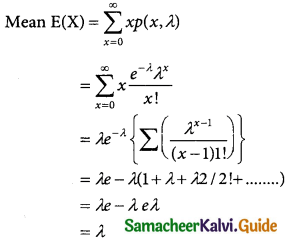Variance (X) = E(X²) – E(X)²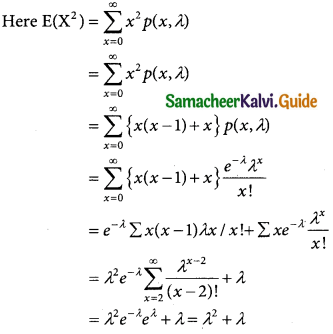Variance (X) = E(X²) – E(X)²
= λ² + λ – (λ)²
= λQuestion 5.
Mention the properties of Poisson distribution.
Solution:

1. Poisson distribution is the only distribution in which the mean and variance are equal.
2. The probability that an event occurs in a given time, distance, area, or volume is the same.

Question 6.
The mortality rate for a certain disease is 7 in 1000. What is the probability for just 2 deaths on account of this disease in a group of 400? Give e(-2.8) = 0.06
Solution:
Since the mortality rate for a certain disease in 7 in loop
∴ p = $$\frac { 7 }{1000}$$ and n = 400
The value of mean A = λp = 400 × $$\frac { 7 }{1000}$$
∴ λ = 2.8
Let x be a random variable following
distribution with p(x) = $$\frac { e^{-λ}λ^x }{x!}$$
∴ the distribution is p(x = 2) = $$\frac { e^{-2-8}(2.8)^2 }{2!}$$
$$\frac { 0.06×7.84 }{2}$$
= 0.2352

Question 7.
Mention the properties of Poisson distribution.
Solution:
Given p = $$\frac{5}{100}$$ = 0.05 and n = 120
⇒ λ = np = (0.05) (120) = 6
Thus X is a Poisson random variable with P (X = x) = $$\frac{e^{-6} 6^{x}}{x !}$$
We want P (no defective bulb) = P (X = 0)
= $$\frac{e^{-6} 6^{0}}{0 !}$$
= e-6
= 0.0025 (Using exponent table)
Thus the probability that a sample of 120 bulbs will not contain any defective bulb is 0.0025.Question 8.
A car hiring firm has two cars. The demand for cars on each day is distributed as a Poisson variate, with a mean of 1.5. Calculate the proportion of days on which
(i) Neither car is used
(ii) Some demand is refused
Solution:
In a poisson distribution n=2
mean λ = 1.5
x follows poison distribution
With in p(x) = $$\frac { e^{-λ}λ^x }{x!}$$
(i) p(neither car is used) = p(x = 0)
$$\frac { e^{-1.5}(1.5)^0 }{0!}$$ = e-1.5
= 0.2231

(ii) p(some demand is refused) = p(x > 2)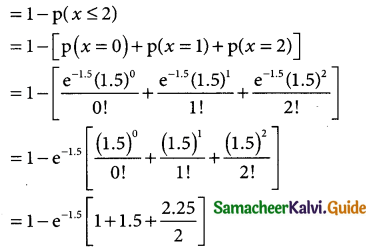= 1 – 0.2231 [1 + 1.5 + 1.125]
= 1 – 0.2231 [3.625]
= 1-0.8087
= 0.1913

Question 9.
The average number of phone calls per minute into the switchboard of a company between 10.00 am and 2.30 pm is 2.5. Find the probability that during one particular minute there will be (i) no phone at all (ii) exactly 3 calls (iii) atleast 5 calls
Solution:
The average number of phone cells per minute into the switch board of a company is λ = 2.5
x follows poisson distribution with(iii) p(atleast 5 calls) = p(x ≥ 5)
= p(x = 5) + p(x = 6) + …………..
= 1 – p(x < 5)Question 10.
The distribution of the number of road accidents pre-day in a city is Poisson with mean 4. Find the number of days out of 100 days when there will be (i) no accident (ii) atleast 2 accidents and (iii) at most 3 accidents.
Solution:
In a possion distribution
mean λ = 4
n = 100
x follows possion distribution with
p(x) = $$\frac { e^{-λ}λ^x }{x!}$$ = $$\frac { e^{-4}(4)^x }{x!}$$
(i) p(no accident) = p(x = 0)
= $$\frac { e^{-4}(4)^0 }{0!}$$ = e-4 = 0.0183
out of 100 days there will be no accident
= n × p(x = 0)
= 100 × 0.0183 = 1.83
= 2 days (approximately)

(ii) p(atleast 2 accidents)
= p(x ≥ 2)
= p(x = 2) + p(x = 3) + p(x = 4) + …………
= 1 – p(x < 2)
= 1 – [p(x = 0) + p(x = 1)]
= 1 – [$$\frac { e^{-4}(4)^0 }{0!}$$ + $$\frac { e^{-4}(4)^1 }{1!}$$]
= 1 – e-4 [l + 4]
= 1 – 0.0183(5) = 1 – 0.0915
= 0.9085
= Out of 100 days there will be atleast 2 accidents = n × p(x ≥ 2)
= 100 × 0.9085
= 90.85
= 91 days (approximately)

(iii) p(atmost 3 accident) = p(x ≤ 3)
= p(x = 0) + p(x = 1) + p(x = 2) + p(x = 3)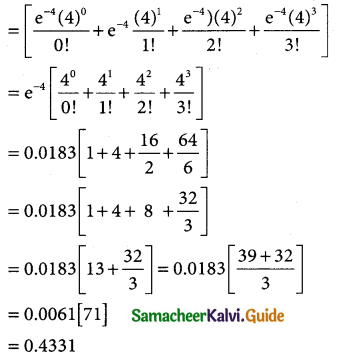out of 100 days there will be at most 3 acccident = n × p(x ≤ 3)
= 100 × 0.4331
= 43.31
= 43 days(approximately)Question 11.
Assuming that a fatal accident in a factory during the year is 1/1200/ calculate the probability that in a factory employing 300 workers there will be atleast two fatal accidents in year, (given e-0.25 = 0.7788 Solution:
Let p be the probability of a fatal accident in a factory during the yeart
p = $$\frac { 1 }{1200}$$ and n = 300 1200
λ = np = 300 × $$\frac { 1 }{1200}$$ = $$\frac { 1 }{4}$$
λ = 0.25
x follows poison distribution with
p(x) = $$\frac { e^{-λ}λ^x }{x!}$$ + $$\frac { e^{-0.25}(0.25) }{x!}$$
p(atleasttwo fatal accidents) = p(x ≥ 2)
= p(x = 2) + p(x = 3) + p(x = 3) + p(x = 4) + ……….
= 1 – p(x < 2)
= 1 – {p(x = 0) + p(x = 1)}= 1 – 0.7788 [1 + 0.25]
= 1 – 0.7788(1.25)
= 1 – 0.9735 = 0.0265
∴ p(x ≥ 2) = 0.0265

Question 12.
The average number of customers, who appear in a counter of a certain bank per minute is two. Find the probability that during a given minute (i) No customer appears (ii) three or more customers appear.
Solution:
The average number of customers ,who appear in a counter of a certain bank per minute = 2
∴ λ = 2
x follows poisson distribution with
p(x) = $$\frac { e^{-λ}λ^x }{x!}$$
x follows poisson distribution with p(x) = $$\frac { e^{-λ}λ^x }{x!}$$
(i) p(no customber appears) = p(x = 0)
= $$\frac { e^{-2}(2)^0 }{0!}$$ = e-2
= 0.1353

(ii) p(three or more customers appears) = p(x ≥ 3)
= p(x = 3) + p{x = 4) + p(x = 5) + ……
= 1 – p(x < 3)
= 1 – {p(x = 0) + p(x = 1) + p(x = 2)}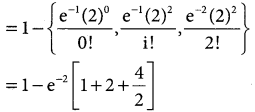= 1 – 2-2 [1 + 2 + 2]
= 1 – 0.1353(5)
= 1 – 0.6765
= 0.3235